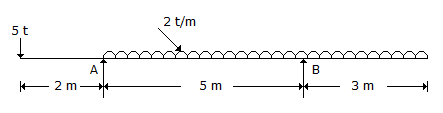# Civil Engineering - Applied Mechanics

### Exercise :: Applied Mechanics - Section 1

16.

The beam shown in below figure is supported by a hinge at A and a roller at B. The reaction RA of the hinged support A of the beam, isA. 10.8 t B. 10.6 t C. 10.4 t D. 10.2 t.

Explanation:

No answer description available for this question. Let us discuss.

17.

The product of mass and velocity of a moving a body, is called

 A. moment B. momentum C. power D. impulse.

Explanation:

No answer description available for this question. Let us discuss.

18.

Engineer's units of force, is

 A. Newton in absolute units B. Dyne in absolute units C. Newton and dyne in absolute units D. All the above.

Explanation:

No answer description available for this question. Let us discuss.

19.

Joule is the unit of

 A. work B. force C. power D. torque E. none of these.

Explanation:

No answer description available for this question. Let us discuss.

20.

The maximum velocity of a body vibrating with a simple harmonic motion of amplitude 150 mm and frequency 2 vibrations/sec, is

 A. 188.5 m/sec B. 18.85 m/sec C. 1.885 m/sec D. 0.18845 m/sec.